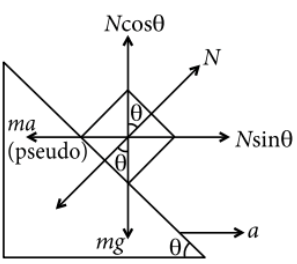Pseudo force
Question

# A block of mass m is placed on a smooth inclined wedge A B C of inclination $\theta$ as shown in the figure. The wedge is given an acceleration a towards the right. The relation between a and $\theta$ for the block to remain stationary on the wedge is

Moderate
Solution

## In non-inertial frame,From (i) and (ii),$\begin{array}{l}\mathrm{tan}\theta =\frac{a}{g}\\ a=g\mathrm{tan}\theta \end{array}$

Get Instant Solutions